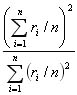### Sparseness of the MUA, SUA and LFP responsesDistribution of sparseness indicies for the MUA (A, B), LFP (C, D) and SUA (E, F) responses. Two definitions of sparseness indices are shown here. In (A, C, E) the sparseness index was defined aswhere ri is the average response to picture i after subtracting the minimum response and there were n pictures (Rolls and Tovee, 1995). In (B, D, F) the sparseness index was defined as the proportion of stimuli that elicited a response that was more than half the maximum response. The arrows indicate the mean values (A= 0.75+/-0.09, B=0.25+/-0.14, C=0.73+/-0.11, D=0.25+/-0.12, E=0.66+/-0.16, F=0.21+/-0.13).

HOME